Our current goal is to find a way to extend our models to accommodate multiple forces simultaneously acting on an object.

Vectors are the perfect tool for doing this.

Consider a 30-kg box (represented as a black square) which is experiencing the forces as shown.

What acceleration results from these three forces acting on our box?

If your intuition is telling you that we need to add these vectors, you'd be right. And adding vectors is something we already know how to do.

We have two means of adding them. We can do it geometrically or algebraically. Let's see both ways.

The red vector is the vector sum of the other three. On graph paper,

it is easy to "complete the triangle" and then use the Pythagorean

Theorem to find the magnitude of our net force.

The direction of our net force is tan-1(30/40) = 36.9° south of east.

Here's our conclusion:

Our box is experiencing multiple forces, but it behaves as if it's experiencing just one - what is called the net force, or total force.  (Net as in the sense of net worth.)

The net force on our box is 50 N at 36.9° south of east.

As the mass of the box is known to be 30-kg, we can easily obtain its acceleration using our model a = F / m.

a = 50 N / 30 kg = 1.67 m/s2 at 36.9° south of east

(Recall that acceleration and force will always have the same direction.)

We will now add the vectors algebraically, which should prove to be simpler.

The net force is the sum of three forces: F1 = 30 j (N) , F2 = -60 j (N) , F3 = 40 i (N)

= (30 j) + (-60 j) + (40 i) = (40 i) + (-30 j)

Now finding the acceleration: a = F / m

This is the answer, yet it is common to sum these two perpendicular vectors, using the Pythagorean Theorem, and report the answer as a single magnitude and direction. The direction comes from using tan-1, as always.

tan-1(1/1.33) = 36.9° south of east

And so our answer is, the box's acceleration is 1.67 m/s2 at 36.9° south of east.

Here's a recommendation. When summing vectors, do it algebraically, BUT draw a quick sketch of the vectors, connected head to tail, along with the resultant vector. Use the sketch do give you a feeling for what their sum should be. Don't worry about making the sketch very precise. (In this example, the sketch should tell you that the net force will be generally southeast.) Realistically, most people quickly progress to mentally combining parallel vectors (parallel vectors will either add, partially cancel, or cancel) and then just sketching the combined or net horizontal force attached head to tail to the net vertical force, along with the resultant vector.

Now, you do one. (And follow my recommendation above.)

An 18-kg box is experiencing the forces as shown below. Find its acceleration.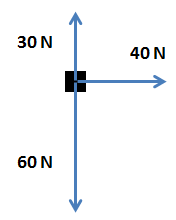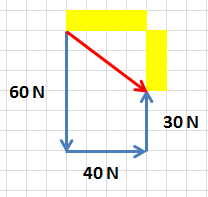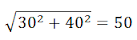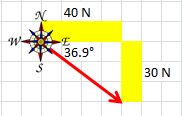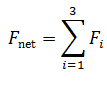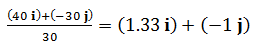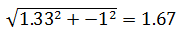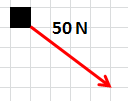The net force on our box.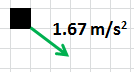The acceleration of our box. (Drawn to a different scale.)

Do not be intimidated by the notation:

The Greek letter "sigma" (Σ) is used by mathematicians and physicists to signify a summation. Here, we are meant to sum forces F1 through F3. If there were 5 forces, we'd change the 3 to a 5. The "i" stands for "index".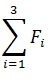I make this look easy, but it's rarely possible to gauge the lengths of the sides of the "triangle" by eye. You'll need a ruler.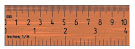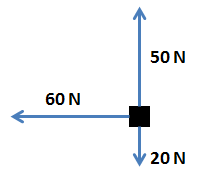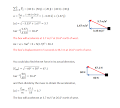The sketch helps you to set up the tan-1 correctly.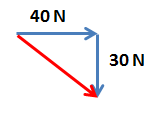Once you have the acceleration, use a kinematic equation (model) to find the displacement (xof the box over (the first) 5 seconds, assuming the box is initially at rest.

Could you handle this situation?

A 50-kg box is experiencing the forces as shown below. Find its acceleration.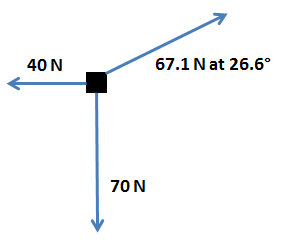A quick sketch results in: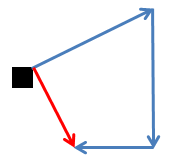The net force should point generally southeast, based on this sketch.

The standard approach to obtaining the net force in a situation like this - where there are vectors that don't align with the axes of the coordinate system - is to resolve the angled vectors into horizontal and vertical components. Upon doing that, you'd proceed exactly as you did for the previous problem. You'd add up all of the horizontal vectors, and separately add all of the vertical vectors, and then you'd add those two vectors: the net horizontal vector to the net vertical vector.

It would look like this:

Here is an diagram in which I've

combined parallel vectors.

Algebraically, the sum of forces looks like this:

As I've shown you through examples, we can now either add the i and j components of the net force, obtain the actual net force with its true direction, and then use a = F / m to find our acceleration ... or we can divide the net force that we have by the mass, obtaining the components of the acceleration. Then, we'd have to add the i and j components of the acceleration to find our actual acceleration, including its direction. It does not matter which approach you choose, unless you are specifically asked to find the actual net force, in which case you must choose the first approach.

I'm going to choose the first approach for this particular problem. Based on the two calculations at left,

the net force is 44.7 N at 63.4° south of east.

Using a = F / m, the acceleration of our box is

a = 44.7 N / 50 kg = 0.89 m/s2 at 63.4° south of east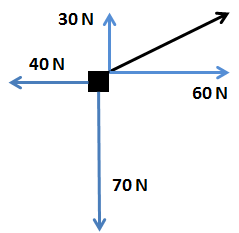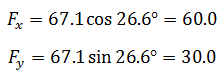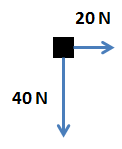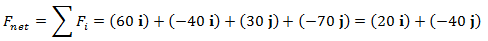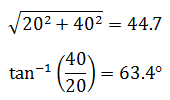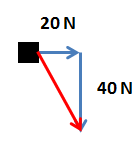Again, you do one.

A 38-kg box is experiencing the forces as shown. Find the net force and its acceleration.

Furthermore, once you have the acceleration, assume the box begins from rest and find its velocity after 6 seconds.

north of east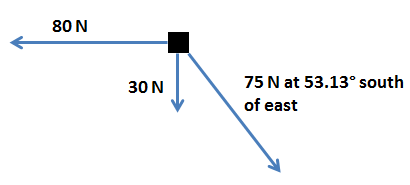Practice  problems

This problem is not much different than Problem 3, involving the Boeing 737, on the last page.

Physics students are often frustrated by apparent "violations" of rules demonstrated only days ago by their teacher.

For example, one day a teacher might treat a falling object as having an acceleration of -9.8 j (m/s2), and then the very next day he or she refers to the acceleration as a positive 9.8 j (m/s2). Isn't downward supposed to be   -j? No, downward is not supposed to be -j. It just happens to be modeled that way most of the time, because physicists find it useful to do so. You might think of the work of devising models as a competition to be the most clever. Think to yourself, is there a more-clever -- that is, easier -- way to model the part of the world I'm focusing on?

When it comes to falling objects, many people find it easier to switch up and down and treat downward as positive j. It's easier because there is no need to write or say "negative" all of the time. I could say, a falling brick has a velocity of 30 j (m/s) and because its acceleration is about 10 j (m/s2), I know that in two seconds it will be traveling at 50 j (m/s). However, you have to ask yourself, would this inversion of up and down still be useful (or make life easier) if the brick had initially been thrown upward? In that case, we'd have to model upward motion as being in the direction of -j, and thus we'd really have gained nothing from the inversion of up and down. Actually, we'd probably have made things worse, because we'd be using a model that made things no easier BUT ALSO was backward to how most people would model this motion. Thus, if someone else examined our work, it would be a bit confusing with no beneficial trade off.

Realize that what is technically happening when someone inverts up and down is simply a rotation of the Cartesian coordinate system.

And yes, you have the power to rotate your coordinate system

however you'd like. Most of the time, there is no benefit to rotating

away from the standard "i point right and j points up" orientation.

But occasionally, rotation of your coordinate system is a very clever

idea.

You may not have realized it, but we have rotated our

coordinate system before. When studying relative motion,

we encountered the following problem.

A spacecraft is headed "forward" (say, toward

Jupiter) at 20,000 m/s, relative to the Earth.

A missile is shot forward from the spacecraft at

400 m/s, relative to the spacecraft. What is the

velocity of the missile, relative to the Earth?

Here, I rotated my coordinate system so that all motion

was along the x-axis.

vi = 20,000 i (m/s)   etc.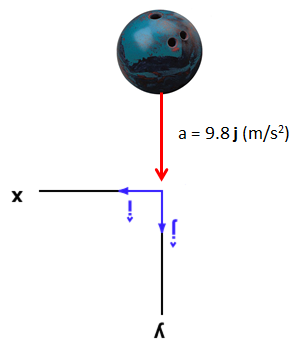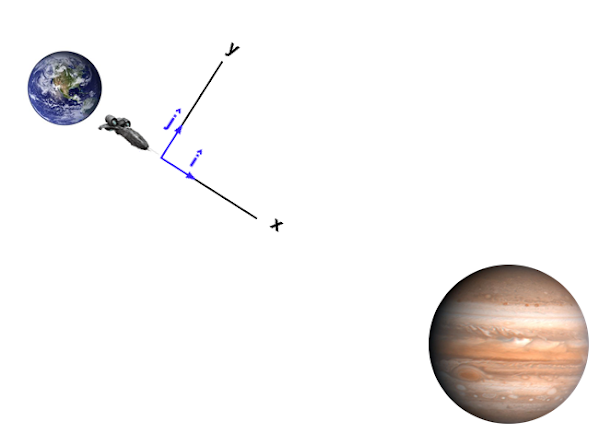Fun Fact: The diameter of Jupiter is about 10 times the diameter of Earth, and about 1/10th the diameter of the Sun. Similar to a star, Jupiter is comprised mostly of hydrogen gas.

Let's now return to a situation in which multiple forces are simultaneously acting on an object. We will find it useful to rotate our coordinate system.

Yes, we could use the standard coordinate system orientation. To sum these vectors, we'd need to resolve both into their components, sum the components, and then re-combine them.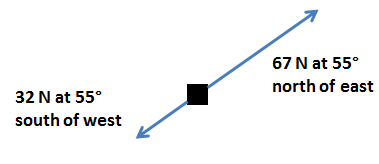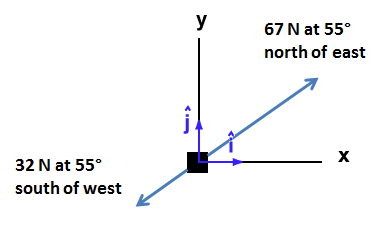However, if you were to realize that the two forces acting here are exactly 180° apart; i.e. pointing in opposite directions, then a rotation of the coordinate system would turn this two-dimensional problem into a simpler one-dimensional problem.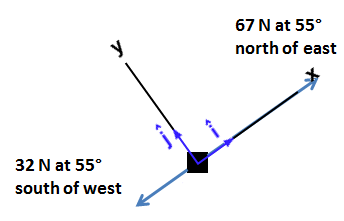We can now quickly sum these two vectors:

The total force is 35 i (N). Or, we might say, 35 N at 55° north of east.

(Actually, if the problem is stated using a particular coordinate system, we should always report our answer in the same coordinate system. We should not report our net force as 35 i because the i we're referring to is not the same i that was implied in the problem. Thus, 35 N at 55° north of east is the proper answer, because it corresponds with the original coordinate system.)

If the mass of the object were 10 kg, it would accelerate at a = F / m = 3.5 m/s2 at 55° north of east.

Later on, you'll have the chance to put this technique into practice when you analyze an object sliding down a hill.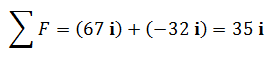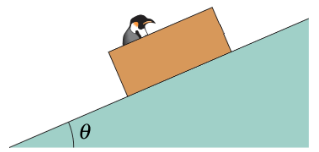Okay, now I'll give you an easy one, in order to make a point.

A multi-ton house is experiencing the forces as shown. Find the net force and its acceleration.

Yes, the net force is simply 0. And so, therefore, is the acceleration.

When you push on the side of your house, and it does not accelerate, this tells you that your force is not the only one. There must be another force that is counteracting yours, preventing acceleration of your house. And aren't you glad!

It is actually quite common for structures to be built such that they can offset external forces, preventing acceleration. The offsetting forces, realize, are not fixed but variable. Thus, if you push on the side of your house with a force of 80 N, an offsetting force of 80 N materializes. And if you push with a force of 200 N, an offsetting force of 200 N materializes. Yet there is a limit, as you very well know. A strong enough force, say from a wrecking ball, can exceed the largest offsetting force the building is capable of generating. And in such a case, the building (or at least a section of it) does accelerate.

Civil engineers are often tasked with making sure that structures do NOT accelerate, regardless of the forces impressed upon them.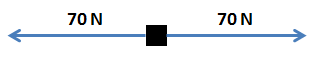Forces

Kinematics